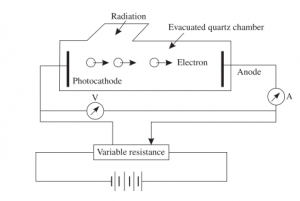# Photoelectric effect – brief introduction

## Photoelectric effect

Photoelectric Effect is the emission of electrons by a substance under the action of light

Photoelectric phenomenon was first noticed by Hertz when he noticed that sparks occur more readily in the air gap of his transmitter when ultraviolet light is directed at one of the metal balls. Hertz, who demonstrated the existence of electromagnetic waves, discovered photoelectric effect but he could understood this effect in terms of the wave model of light.This figure given above is a schematic arrangement to study photoelectric effect. The experiment consists of an evacuated tube containing two electrodes connected to a source of variable voltage. The light  radiation is allowed to enter the jacket through a quartz window.The radiation falls on the electrode A, called cathode. The electrode B can be kept at positive or negative potential with respect to the cathode. A sensitive ammeter is put in the circuit to record the current resulting from the photo-electrons. The potential difference between the cathode and the anode can be measured by voltmeter.

Now when light is made to fall on clean metal surface (that is cathode) electrons are emitted from the metal surface. If some of the emitted electrons reach the anode then current begin to flow in the circuit and can be recorded via ammeter. The number of the
emitted electrons reaching the anode can be increased or decreased by making the anode positive or negative with respect to the cathode.

1. For a constant potential difference between the cathode and anode, the number of electrons emitted from cathode  increases with increasing intensity of radiation.
2. For a constant intensity and frequency of incident radiation the photoelectric current varies with the potential difference V between the cathode and anode and reaches a constant value beyond which further increase of potential difference dose not effect the photoelectric current, on the other hand, if the plate B is made more and more negative with respect of the photo-cathode surface the current decreases. This negative potential (with respect to cathode) of the plate is called retarding potential. For a particular value of retarding potential, the photoelectric current becomes zero. This potential is called cut-off or stopping potential $V_{0}$ and is measure of maximum kinetic energy of photo-electrons and we can write
$T_{max} = eV_{0}$
where $T_{max}$ is the maximum kinetic energy of the ejected electron.
3. The stopping potential $V_{0}$ and hence the maximum kinetic energy $T_{max}$ of photo-electrons is independent of the intensity of incident radiation and depends only on the frequency $\nu$ of radiation.
4. For each substance there exists a characteristic frequency $\nu_{0}$ such that for radiation with frequency below $\nu_{0}$ the photo-electrons are not ejected from the surface. This frequency is called the threshold frequency and the corresponding wavelength is called threshold wavelength, $\lambda_{0}$ .
5. It has been observed that as soon as the light is incident on the substance, the electrons are emitted i.e., there is no time lag between the incidence of radiation and the ejection of electron.

Failure of classical physics

The phenomenon of photoelectric effect cannot be understood by using wave model of radiation. In classical theory, light consists of oscillating electric and magnetic fields; here the intensity of radiation is proportional to the square of the electric vector E. The force on electron exerted by incident radiation is eE and hence the kinetic energy of ejected electron should depend on the intensity of the radiation but the experimental results does not agree with the classical physics predictions. Also the existence of threshold frequency has no explanation at all in the classical theory. Also according to classical theory there should be considerable time lag between the arrival of the radiation and the ejection of electron, which is contrary to the observation.

Explanation of photo-electric effect

Albert Einstein first proposed the satisfactory explanation of this phenomenon in 1905. Einstein proposed that radiation energy is not continuously distributed over the wave-front, but the light energy consists of discrete quanta of energy $h\nu$ called a photon, that penetrates the surface of the cathode, all of its energy is given completely to an electron . The photon’s energy would be associated with its frequency $(\nu)$, through a proportionality constant known as Planck’s constant $(h)$, or alternately, using the wavelength $(\lambda)$ and the speed of light $(c)$:

$E = h\nu = hc / \lambda$
or the momentum equation:
$p = h / \lambda$
When a photon is incident on the surface of a material, some of its energy is spent in making the electron free and the rest appears as kinetic energy of the electron. The electrons at the surface of the material are most loosely bound and require minimum energy for their liberation. This energy is called the work function $W_{0}$ of the material. The maximum kinetic energy of photo electrons ejected from the surface is given by
$T_{max}=h\nu – W_{0}$

So, work function, is the minimum energy that must be supplied to the electron for it to leave the metal. The prediction is that there exists a minimum frequency that is independent of the intensity of light, below which electrons cannot be ejected from the metal. This proposal was proved by Millikan when in 1914 he published his results of the voltage required to stop electrons ejected from a metal by light of frequency $\nu$.

Einstein was awarded the 1921 Nobel prize in physics (which he received in 1922) for his work on the photoelectric effect .

Subscribe
Notify ofThis site uses Akismet to reduce spam. Learn how your comment data is processed.